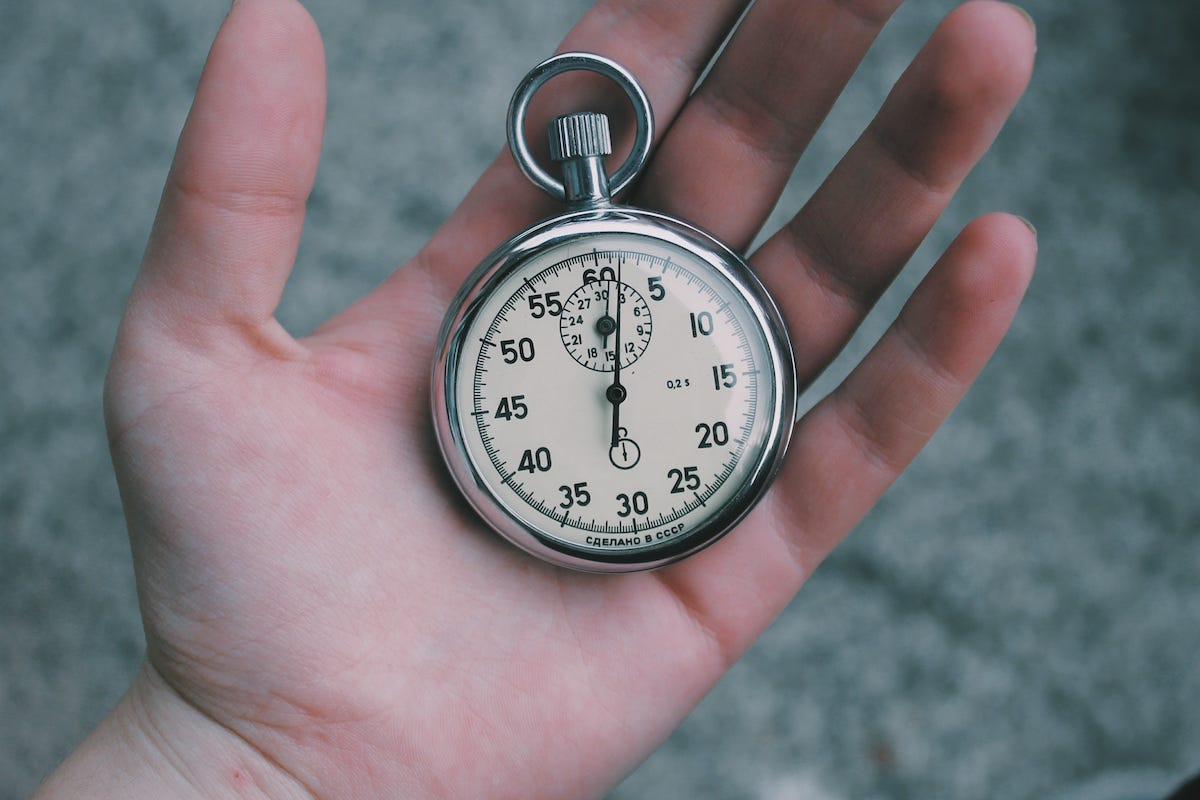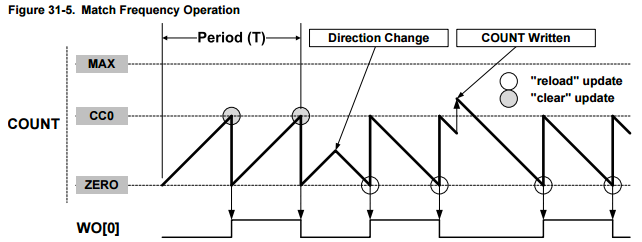Veri Ivanova

# Smoothly Changing a Timer’s Frequency on the Arduino Zero

Here’s what I set out to do:

1. Setup a basic timer using the Arduino Zero.
2. Use a potentiometer to control the frequency of that timer.
3. Profit.

Easy right? … not quite.

Jump to the code: github.com/nebs/arduino-zero-timer-demo

#### Project Overview

The demo project is composed of an Arduino Zero, an LED and a potentiometer.

The LED flashes in sync with the timer. The potentiometer controls the frequency of the timer.

#### Useful Resources

If you’re new to Arduino Zero timers I’d recommend taking a look at the following resources:

• SAM D21 DataSheet. This is the data sheet for the Arduino Zero’s microcontroller chip. Study chapter 30.
• Adafruit ZeroTimer. I haven’t actually used this library but it served as a nice reference and sanity check.
• Max Bader’s Arduino Tools. A big thank you to Max for posting his code. This helped me get to 80% of where I wanted to go.
• Official Arduino Zero Forum. It’s a little quiet there, but it’s a nice resource to have nonetheless.

#### Basic Timer Setup

The SAM D21 microcontroller has multiple timers each of which can be used for different tasks and configured in numerous ways. I was only concerned with a very simple use case: Setting up a single timer that fires an interrupt at a chosen frequency.

Setting up a timer is “simply” a matter of flipping bits and updating registers. That’s easier said than done. The hardest part is making sense of the different timer modes and strange register names.

Thankfully, most of the boilerplate is fairly simple for basic timer operation. I will refer you to the code for that. The more interesting part is setting the timer’s frequency.

In order to have a timer tick at a specific frequency we need a way to set its period. One way to do this is to have the timer reset its counter to zero whenever it reaches some value. This “some value” is the capture/compare register (CCx).

Normally the timer counts up to MAX before jumping back to 0. But we want it to count to our compare register instead of MAX. In order to do that we set the Waveform Output Generation configuration to the “match” mode :

`TC->CTRLA.reg |= TC_CTRLA_WAVEGEN_MFRQ;`

As can be seen in Figure 31–5 below, in match mode, the counter jumps to 0 when it reaches the value set in CC0.SAM D21 Datasheet (p. 688)

In order to use the compare register in a useful manner we need a way to compute its value from the intended timer’s frequency (Hz).

By default the timer’s counter increments by 1 every CPU clock cycle. On the Arduino Zero that’s 48MHz. For example, in order to get a 1Hz timer we would have to set the compare register to 47,999,999 (since the counter starts at 0). Unfortunately this is not possible since in 16-bit mode the maximum value we could set is 2¹⁶ = 65,536. Prescalers to the rescue!

A prescaler is basically a clock divider. The available prescalers are: 1, 2, 4, 8, 16, 64, 256, 1024. For example, if you choose 1024, the timer clock effectively becomes 48Mhz / 1024 = 46.875KHz. So in this case the compare register would be 46,874 (which can now be represented using 16 bits).

More generally, given the prescaler, CPU frequency and desired timer frequency, you can find the compare register value using this formula:

`Compare Register = [CPU_Hz / (Prescaler * TimerFreq_Hz)] - 1`

#### Timer Frequency Modulation

The next part of the project involved finding a way to control the timer’s frequency using a potentiometer.

My first approach was to simply read the potentiometer value and update the timer’s compare register directly:

`int frequencyHz = (analogRead(POT_PIN) / 30) + 1int compareValue = (CPU_HZ / (TIMER_PRESCALER_DIV * frequencyHz)) — 1;`
`TcCount16* TC = (TcCount16*) TC3;TC->CC.reg = compareValue;while (TC->STATUS.bit.SYNCBUSY == 1);`

This turned out to be quite buggy.

Problem #1

When turning the potentiometer I expected the LED to change its flashing speed smoothly. Instead, it appeared to freeze and flash unexpectedly.

My first guess was that the potentiometer was jittery. To solve this I wrapped the frequency setting inside a threshold guard so that we’d only set it if the potentiometer value changed significantly since the last read.

`int newPotValue = analogRead(POT_PIN);if (abs(newPotValue — potValue) > POT_JITTER_THRESHOLD) {  potValue = newPotValue;  setTimerFrequency(frequencyFromPotValue(potValue));}`

This seemed to improve things a little bit.

Problem #2

The LED still flickered as I swept the potentiometer.

My next thought was that perhaps some of the glitches were caused by the compare register being written while the counter value was in a relatively unusual state. For example if the compare register was suddenly set to a value below the counter.

So I updated the code to reset the timer’s counter to 0 whenever I updated the compare register.

`int frequencyHz = (analogRead(POT_PIN) / 30) + 1int compareValue = (CPU_HZ / (TIMER_PRESCALER_DIV * frequencyHz)) — 1;`
`TcCount16* TC = (TcCount16*) TC3;TC->COUNT.reg = 0;TC->CC.reg = compareValue;while (TC->STATUS.bit.SYNCBUSY == 1);`

At this point I was getting close.

Problem #3

Unfortunately there were still random LED flickers when I swept the potentiometer. They were less abrupt this time, and the flashing would eventually continue at the correct rate, but the transition from one frequency to another wasn’t smooth.

After staring at this problem for a while it occurred to me that perhaps the flickers were caused by the counter jumping suddenly to 0. To fix this I tried mapping the new counter value relative to the old one’s position.

For example let’s assume the compare register was 200 and the counter was at 100 (half way). Before updating the compare register I would ensure that the new counter value was set to the same relative spot (in this case, half way).

`int compareValue = (CPU_HZ / (TIMER_PRESCALER_DIV * frequencyHz)) — 1;TcCount16* TC = (TcCount16*) TC3;TC->COUNT.reg = map(TC->COUNT.reg, 0, TC->CC.reg, 0, compareValue);TC->CC.reg = compareValue;while (TC->STATUS.bit.SYNCBUSY == 1);`

Sweeping the potentiometer now resulted in a smooth gradual change of the timer frequency with no glitches or flickers of the LED. Success!

#### Conclusion

Learning about the Arduino Zero’s timers was both a frustrating and rewarding experience. I‘m no expert and I have much to learn but I hope this post and code will help someone out in the future.

Download the code: github.com/nebs/arduino-zero-timer-demo